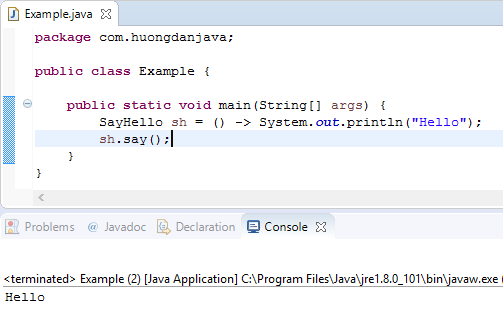Lambda Expression was introduced to enable functional programming in Java, help us can remove unnecessary code. So, what is Lambda Expression? In this tutorial, I will introduce to you all some basic knowledges about Lambda Expression in Java.

OK, let’s go.

### Why do we need Lambda Expression?

To answer this question, I will give you an example.

Assume, I have an interface, named SayHello with content as below:

Now, I want to use this interface to implement an object which can print the text “Hello” in my application. Before Java 8, I have some approachs as below:

• The first one, I will create a class implement interface SayHello:

and using it in my application

• The second one, I can define an anonymous class in the main method of my application.

Do you think with 2 approaches above, we have many lines of code need to be implemented? The first one, we must create new class to implement interface SayHello, and the second one, we must implement method say() in the main method of Example class. Meanwhile, the only thing we need, only print the text “Hello”. In other words, we only need the line as below:

To resolve this problem, from Java 8, Lambda Expression was introduced. With Lambda Expression, our code will simple as below:

Result:Here, () -> System.out.println(“Hello”), this is a Lambda Expression.

### How to use Lambda Expression?

Lambda Expression is an anonymous function, include 2 parts:

• The first part lays before the signal “->” to declare the arguments. These arguments will lay between the signal “(” and “)” and each arguments will be separated by a comma. In case, we only have one argument, we can remove the signal “(” and “)”.
• The last part after the signal “->” will be a block execution code. This code block will lay between the signal “{” and “}”. In case the block code has only one line, we can remove “{” and “}” and if it has return statement, we can remove return statement also.

Lambda Expression will use with a Functional Interface which only has one abstract method. This abstract method can have no-argument or more arguments.

Above example is the way to work with interface that has abstract method without argument.

With the interface has abstract method with one or more arguments, for example:

we can declare Lambda Expression as below:

Result: